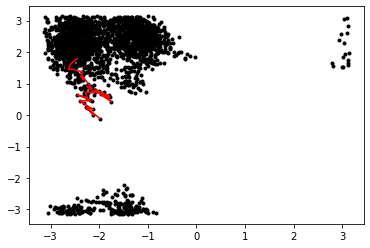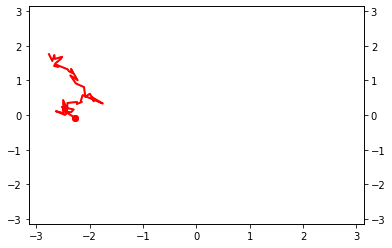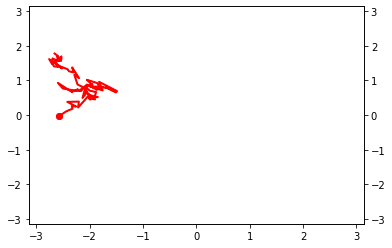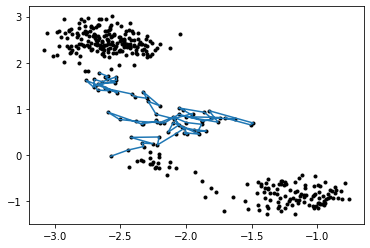# Fixed Length TPS on Alanine Dipeptide

Although fixed length TPS was developed before flexible length TPS, it is actually a little harder to set up. It is also less efficient, so under most circumstances it is better to use flexible-length TPS.

Note that the first notebook here is the same as is used in the flexible length TPS example. In addition, the notebooks to run the simulation and to analyze it are also very similar to the flexible length example.

# Obtaining an equilibrated initial trajectory¶

This is the first file to run in the alanine dipeptide TPS example. This teaches you how to:

• set up an engine using OpenMM
• set up states using MDTraj-based collective variables
• obtain a initial trajectory using high temperature MD
• equilibrate by using shooting moves until the first decorrelated trajectory

We assume at this point that you are familiar with the basic concepts of OPS. If you find this file confusing, we recommend working through the toy model examples.

In :
from __future__ import print_function
%matplotlib inline
import matplotlib.pyplot as plt
import openpathsampling as paths

import openpathsampling.engines.openmm as omm
from simtk.openmm import app
import simtk.openmm as mm
import simtk.unit as unit
from openmmtools.integrators import VVVRIntegrator

import mdtraj as md

import numpy as np

import os


## Setting up high-temperature engine¶

Now we set things up for the OpenMM simulation. We will need a openmm.System object and an openmm.Integrator object.

To learn more about OpenMM, read the OpenMM documentation. The code we use here is based on output from the convenient web-based OpenMM builder.

In :
# this cell is all OpenMM specific
forcefield = app.ForceField('amber96.xml', 'tip3p.xml')
pdb = app.PDBFile(initial_pdb)
system = forcefield.createSystem(
pdb.topology,
nonbondedMethod=app.PME,
nonbondedCutoff=1.0*unit.nanometers,
constraints=app.HBonds,
rigidWater=True,
ewaldErrorTolerance=0.0005
)
hi_T_integrator = VVVRIntegrator(
500*unit.kelvin,
1.0/unit.picoseconds,
2.0*unit.femtoseconds)
hi_T_integrator.setConstraintTolerance(0.00001)


The storage file will need a template snapshot. In addition, the OPS OpenMM-based Engine has a few properties and options that are set by these dictionaries.

In :
template = omm.snapshot_from_pdb(initial_pdb)
openmm_properties = {'OpenCLPrecision': 'mixed'}
engine_options = {
'n_steps_per_frame': 10,
'n_frames_max': 20000
}

In :
hi_T_engine = omm.Engine(
template.topology,
system,
hi_T_integrator,
openmm_properties=openmm_properties,
options=engine_options
)
hi_T_engine.name = '500K'

In :
hi_T_engine.current_snapshot = template
hi_T_engine.minimize()


## Defining states¶

First we define the CVs using the md.compute_dihedrals function. Then we define our states using PeriodicCVDefinedVolume (since our CVs are periodic.)

In :
# define the CVs
psi = paths.MDTrajFunctionCV(
"psi", md.compute_dihedrals,
topology=template.topology, indices=[[6,8,14,16]]
).enable_diskcache()
phi = paths.MDTrajFunctionCV(
"phi", md.compute_dihedrals,
topology=template.topology, indices=[[4,6,8,14]]
).enable_diskcache()

In :
# define the states
deg = 180.0/np.pi
C_7eq = (
paths.PeriodicCVDefinedVolume(phi, lambda_min=-180/deg, lambda_max=0/deg,
period_min=-np.pi, period_max=np.pi) &
paths.PeriodicCVDefinedVolume(psi, lambda_min=100/deg, lambda_max=200/deg,
period_min=-np.pi, period_max=np.pi)
).named("C_7eq")
# similarly, without bothering with the labels:
alpha_R = (
paths.PeriodicCVDefinedVolume(phi, -180/deg, 0/deg, -np.pi, np.pi) &
paths.PeriodicCVDefinedVolume(psi, -100/deg, 0/deg, -np.pi, np.pi)
).named("alpha_R")


## Getting a first trajectory¶

We'll use the VisitAllStatesEnsemble to create a high-temperature trajectory that visits all of our states. Here we only have 2 states, but this approach generalizes to multiple states.

In :
visit_all = paths.VisitAllStatesEnsemble([C_7eq, alpha_R])
trajectory = hi_T_engine.generate(hi_T_engine.current_snapshot, [visit_all.can_append])

Ran 2143 frames. Found states [alpha_R,C_7eq]. Looking for [].


## Plotting the trajectory¶

In :
# create a network so we can use its ensemble to obtain an initial trajectory
# use all-to-all because initial traj can be A->B or B->A; will be reversed
tmp_network = paths.TPSNetwork.from_states_all_to_all([C_7eq, alpha_R])

In :
# take the subtrajectory matching the ensemble (only one ensemble, only one subtraj)
subtrajectories = []
for ens in tmp_network.analysis_ensembles:
subtrajectories += ens.split(trajectory)
print(subtrajectories)

[Trajectory]

In :
plt.plot(phi(trajectory), psi(trajectory), 'k.')
plt.plot(phi(subtrajectories), psi(subtrajectories), 'r')

Out:
[<matplotlib.lines.Line2D at 0x151a20509ad0>]## Setting up the normal temperature engine¶

We'll create another engine that uses a 300K integrator, and equilibrate to a 300K path from the 500K path.

In :
integrator = VVVRIntegrator(
300*unit.kelvin,
1.0/unit.picoseconds,
2.0*unit.femtoseconds
)
integrator.setConstraintTolerance(0.00001)
engine = omm.Engine(
template.topology,
system,
integrator,
openmm_properties=openmm_properties,
options=engine_options
).named('300K')


## Using TPS to equilibrate¶

This is, again, a simple path sampling setup. We use the same TPSNetwork we'll use later, and only shooting moves. One the initial conditions are correctly set up, we run one step at a time until the initial trajectory is decorrelated.

This setup of a path sampler always consists of defining a network and a move_scheme. See toy model notebooks for further discussion.

In :
network = paths.TPSNetwork(initial_states=C_7eq,
final_states=alpha_R).named('tps_network')
scheme = paths.OneWayShootingMoveScheme(network,
selector=paths.UniformSelector(),
engine=engine).named('equil_scheme')


The move scheme's initial_conditions_from_trajectories method will take whatever input you give it and try to generate valid initial conditions. This includes looking for subtrajectories that satisfy the ensembles you're sampling, as well as reversing the trajectory. If it succeeds, you'll see the message "No missing ensembles. No extra ensembles." It's also a good practice to use scheme.assert_initial_conditions to ensure that your initial conditions are valid. This will halt a script if the initial conditions are not valid.

In :
# make subtrajectories into initial conditions (trajectories become a sampleset)
initial_conditions = scheme.initial_conditions_from_trajectories(subtrajectories)

No missing ensembles.
No extra ensembles.

In :
# check that initial conditions are valid and complete (raise AssertionError otherwise)
scheme.assert_initial_conditions(initial_conditions)


We can create a StepVisualizer2D, which plays the simulation as it is running. It requires CVs for the $x$ direction and the $y$ direction, as well as bounds of the plot. You can set the background attribute to an existing matplotlib Figure, and the simulation will plot on top of that. See the toy model MSTIS example for an example of that.

This isn't necessary, and isn't even a useful for long simulations (which typically won't be in an interactive notebook), but it can be fun!

In :
visualizer = paths.StepVisualizer2D(network, phi, psi, xlim=[-3.14, 3.14],
ylim=[-3.14, 3.14])

In :
sampler = paths.PathSampling(storage=paths.Storage("ad_tps_equil.nc", "w", template),
move_scheme=scheme,
sample_set=initial_conditions)
sampler.live_visualizer = visualizer

In :
# initially, these trajectories are correlated (actually, identical)
# once decorrelated, we have a (somewhat) reasonable 300K trajectory
initial_conditions.trajectory.is_correlated(sampler.sample_set.trajectory)

Out:
True
In :
sampler.run_until_decorrelated()Step 6: All trajectories decorrelated!

In :
# now we have decorrelated: no frames are in common
initial_conditions.trajectory.is_correlated(sampler.sample_set.trajectory)

Out:
False
In :
# run an extra 10 to decorrelate a little futher
sampler.run(10)DONE! Completed 15 Monte Carlo cycles.

In :
sampler.storage.close()


From here, you can either extend this to a longer trajectory for the fixed length TPS in the AD_tps_1b_trajectory.ipynb notebook, or go straight to flexible length TPS in the AD_tps_2a_run_flex.ipynb notebook.

# Obtaining an initial trajectory for fixed-length TPS¶

This notebook is part of the fixed length TPS example. It requires the file ad_tps_equil.nc, which is written in the notebook AD_tps_1_trajectory.ipynb.

In this notebook, you will learn:

• how to set up a FixedLengthTPSNetwork
• how to extend a transition path to satisfy the fixed length TPS ensemble
• how to save specific objects to a file
In :
from __future__ import print_function
%matplotlib inline
import matplotlib.pyplot as plt

import openpathsampling as paths


First, we open the file we made in AD_tps_1_trajectory.ipynb and load various things we need from that.

In :
old_storage = paths.Storage("ad_tps_equil.nc", "r")

In :
engine = old_storage.engines['300K']
C_7eq = old_storage.volumes['C_7eq']
alpha_R = old_storage.volumes['alpha_R']
traj = old_storage.samplesets[-1].trajectory

phi = old_storage.cvs['phi']
psi = old_storage.cvs['psi']


## Building a trajectory to suit the ensemble¶

We're starting from a trajectory that makes the transition. However, we need that trajectory to be longer than it is.

There's an important subtlety here: we can't just extend the trajectory in one direction until is satisfies our length requirement, because it is very possible that the final frame would be in the no-man's-land that isn't either state, and then it wouldn't satisfy the ensemble. (Additionally, without a shifting move, having the transition at the far edge of the trajectory time could be problematic.)

So our approach here is to extend the trajectory in either direction by half the fixed length. That gives us a total trajectory length of the fixed length plus the length of the original trajectory. Within this trajectory, we try to find an subtrajectory that satisfies our ensemble. If we don't, then we add more frames to each side and try again.

In :
network = paths.FixedLengthTPSNetwork(C_7eq, alpha_R,
length=400).named('fixed_tps_network')

In :
trajectories = []
i=0
while len(trajectories) == 0 and i < 5:
max_len = 200 + i*50
fwd_traj = engine.generate(traj[-1], [lambda traj, foo: len(traj) < max_len])
bkwd_traj = engine.generate(traj, [lambda traj, foo: len(traj) < max_len], direction=-1)
new_traj = bkwd_traj[:-1] + traj + fwd_traj[1:]
trajectories = network.sampling_ensembles.split(new_traj)
print(trajectories)

/scratch/dswenson/miniconda/envs/dev/lib/python3.7/site-packages/mdtraj/utils/validation.py:116: TypeCastPerformanceWarning: Casting unitcell_vectors dtype=float64 to <class 'numpy.float32'>
TypeCastPerformanceWarning)

[Trajectory]

In :
# raises an error if we still haven't found a suitable trajectory
trajectory = trajectories


## Plot the trajectory¶

This is exactly as done in AD_tps_1_trajectory.ipynb.

In :
plt.plot(phi(trajectory), psi(trajectory), '.k')
plt.plot(phi(traj), psi(traj))

Out:
[<matplotlib.lines.Line2D at 0x148248e591d0>]## Save stuff¶

When we do path sampling, the PathSampling object automatically handles saving for us. However, we can also save things explicitly. If you save an object that contains another object, the inner object will be saved. For example, saving the network will also save both of the stable states (which, in turn, save the CVs).

In :
storage = paths.Storage("ad_fixed_tps_traj.nc", "w")

In :
storage.save(trajectory)
storage.save(network)
storage.save(engine);  # not technically required, saved with trajectory

In :
storage.close()
old_storage.close()


# Running a fixed-length TPS simulation¶

This is file runs the main calculation for the fixed length TPS simulation. It requires the file ad_fixed_tps_traj.nc, which is written in the notebook AD_tps_1b_trajectory.ipynb.

In this file, you will learn:

• how to set up and run a fixed length TPS simulation

NB: This is a long calculation. In practice, it would be best to export the Python from this notebook, remove the live_visualizer, and run non-interactively on a computing node.

In :
from __future__ import print_function
import openpathsampling as paths


## Load engine, trajectory, and states from file¶

In :
old_storage = paths.Storage("ad_fixed_tps_traj.nc", "r")

In :
engine = old_storage.engines['300K']
network = old_storage.networks['fixed_tps_network']
traj = old_storage.trajectories


## TPS¶

The only difference between this and the flexible path length example in AD_tps_2a_run_flex.ipynb is that we used a FixedLengthTPSNetwork. We selected the length=400 (8 ps) as a maximum length based on the results from a flexible path length run.

In :
scheme = paths.OneWayShootingMoveScheme(network,
selector=paths.UniformSelector(),
engine=engine)

In :
initial_conditions = scheme.initial_conditions_from_trajectories(traj)

No missing ensembles.
No extra ensembles.

In :
storage = paths.Storage("ad_fixed_tps.nc", "w")
storage.save(initial_conditions);  # save these to give storage a template

In :
sampler = paths.PathSampling(storage=storage,
move_scheme=scheme,
sample_set=initial_conditions)

In :
sampler.run(10000)

Working on Monte Carlo cycle number 10000
Running for 10 hours 43 minutes 11 seconds -  3.86 seconds per step
Estimated time remaining: 3 seconds
DONE! Completed 10000 Monte Carlo cycles.

In :
old_storage.close()
storage.close()


With this done, you can go on to do the fixed-length parts of the analysis in AD_tps_3b_analysis_fixed.ipynb.

In [ ]:



# Analyzing the fixed path length simulation¶

We start with the same sorts of analysis as we did in the flexible path length example: we get an overview of the file, then we look at the acceptance ratio, and then we look at the move history tree and the decorrelated trajectories.

In :
from __future__ import print_function
%matplotlib inline
import openpathsampling as paths
import numpy as np
import matplotlib.pyplot as plt
import os
import openpathsampling.visualize as ops_vis
from IPython.display import SVG

In :
# note that this log will overwrite the log from the previous notebook
#import logging.config
#logging.config.fileConfig("../resources/logging.conf", disable_existing_loggers=False)

In :
%%time

CPU times: user 40.2 s, sys: 4.36 s, total: 44.5 s
Wall time: 45 s

In :
engine = fixed.engines
fixed_scheme = fixed.schemes

print("File size: {0} for {1} steps, {2} snapshots".format(
fixed.file_size_str,
len(fixed.steps),
len(fixed.snapshots)
))

File size: 74.98GB for 10001 steps, 3985370 snapshots

In :
fixed_scheme.move_summary(fixed.steps)

shooting ran 100.000% (expected 100.00%) of the cycles with acceptance 5045/10000 (50.45%)

In :
history = ops_vis.PathTree(
fixed.steps[0:25],
ops_vis.ReplicaEvolution(
replica=0
)
)
print(len(list(history.samples)))

SVG(history.svg())

15

Out:
In :
history.options.css['scale_x'] = 1
SVG(history.svg())

Out:
In :
with open("fixed_tps_tree.svg", 'w') as svg_out:
svg_out.write(history.svg())

In :
print("Decorrelated trajectories:", len(history.generator.decorrelated_trajectories))

Decorrelated trajectories: 2

In :
full_history = ops_vis.PathTree(
fixed.steps,
ops_vis.ReplicaEvolution(
replica=0
)
)

n_decorrelated = len(full_history.generator.decorrelated_trajectories)

print("All decorrelated trajectories:", n_decorrelated)

All decorrelated trajectories: 443


Note that the number of MC steps (and even more so, time steps) per decorrelated trajectory is much higher than in the case of flexible path length TPS. This is the heart of the argument that flexible path length approaches are more efficient than fixed path length approaches: with a fixed path length, it takes much more effort to get a decorrelated trajectory.

In [ ]: